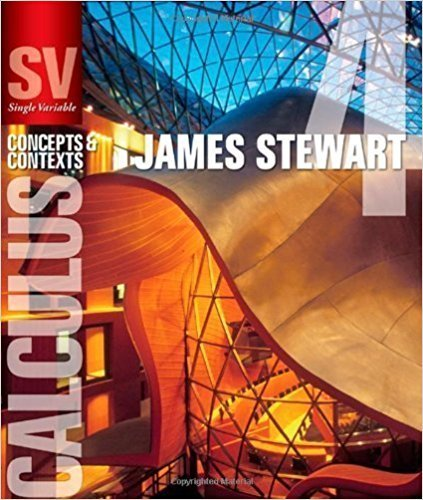×
×

# Solutions for Chapter 5.3: EVALUATING DEFINITE INTEGRALS## Full solutions for Single Variable Calculus: Concepts and Contexts (Stewart's Calculus Series) | 4th Edition

ISBN: 9780495559726Solutions for Chapter 5.3: EVALUATING DEFINITE INTEGRALS

Solutions for Chapter 5.3
4 5 0 383 Reviews
10
2
##### ISBN: 9780495559726

Chapter 5.3: EVALUATING DEFINITE INTEGRALS includes 76 full step-by-step solutions. Single Variable Calculus: Concepts and Contexts (Stewart's Calculus Series) was written by and is associated to the ISBN: 9780495559726. This expansive textbook survival guide covers the following chapters and their solutions. Since 76 problems in chapter 5.3: EVALUATING DEFINITE INTEGRALS have been answered, more than 20480 students have viewed full step-by-step solutions from this chapter. This textbook survival guide was created for the textbook: Single Variable Calculus: Concepts and Contexts (Stewart's Calculus Series), edition: 4.

Key Calculus Terms and definitions covered in this textbook
• Bias

A flaw in the design of a sampling process that systematically causes the sample to differ from the population with respect to the statistic being measured. Undercoverage bias results when the sample systematically excludes one or more segments of the population. Voluntary response bias results when a sample consists only of those who volunteer their responses. Response bias results when the sampling design intentionally or unintentionally influences the responses

• Compounded k times per year

Interest compounded using the formula A = Pa1 + rkbkt where k = 1 is compounded annually, k = 4 is compounded quarterly k = 12 is compounded monthly, etc.

• Convergence of a sequence

A sequence {an} converges to a if limn: q an = a

• Convergence of a series

A series aqk=1 ak converges to a sum S if imn: q ank=1ak = S

• Cosine

The function y = cos x

• De Moivre’s theorem

(r(cos ? + i sin ?))n = r n (cos n? + i sin n?)

• Equally likely outcomes

Outcomes of an experiment that have the same probability of occurring.

• Grapher or graphing utility

Graphing calculator or a computer with graphing software.

• Higher-degree polynomial function

A polynomial function whose degree is ? 3

• Magnitude of a real number

See Absolute value of a real number

• Origin

The number zero on a number line, or the point where the x- and y-axes cross in the Cartesian coordinate system, or the point where the x-, y-, and z-axes cross in Cartesian three-dimensional space

• Projectile motion

The movement of an object that is subject only to the force of gravity

• Reciprocal function

The function ƒ(x) = 1x

• Remainder theorem

If a polynomial f(x) is divided by x - c , the remainder is ƒ(c)

• Replication

The principle of experimental design that minimizes the effects of chance variation by repeating the experiment multiple times.

• Secant

The function y = sec x.

• Semiminor axis

The distance from the center of an ellipse to a point on the ellipse along a line perpendicular to the major axis.

• Third quartile

See Quartile.

• Time plot

A line graph in which time is measured on the horizontal axis.

• Work

The product of a force applied to an object over a given distance W = ƒFƒ ƒAB!ƒ.

×